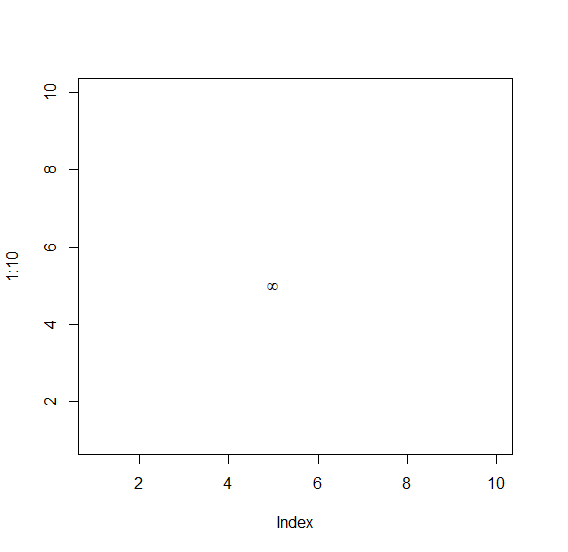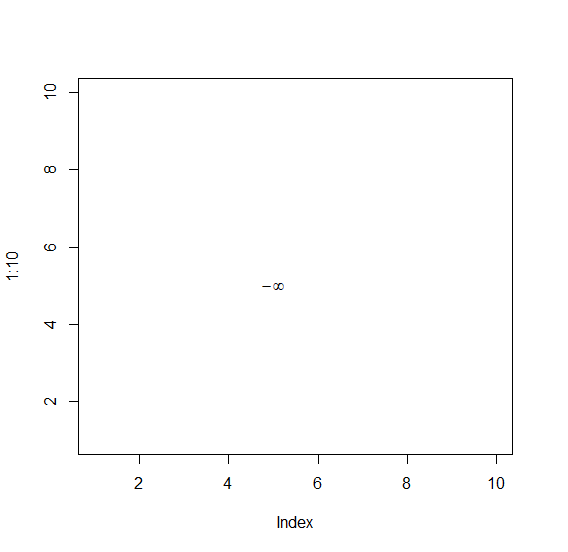# How to display infinity symbol in base R plot?

To display infinity symbol in base R plot, we can use text function and expression function. Inside expression function we can put infinity word for the display of infinity. For Example, if we want to display infinity at position X=2 and Y=4 then we can use the below mentioned command −

text(2,4,expression(infinity))

## Example 1

To display infinity symbol in base R plot, use the snippet given below −

plot(1:10,type="n")
text(5,5,expression(infinity))

## Output

If you execute the above given snippet, it generates the following Output −## Example 2

To display infinity symbol in base R plot, use the snippet given below −

plot(1:10,type="n")
text(5,5,expression(-infinity))

## Output

If you execute the above given snippet, it generates the following Output −